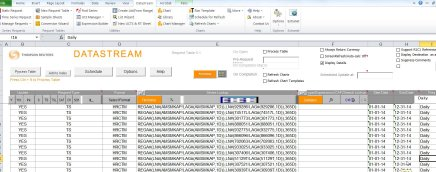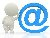## Abnormal Return & market choiceToday I had a student who wanted the abnormal return for a couple of lists of stocks but wanted to choose different markets depending on the stock. To get the Abnormal Return from datastream you can use the following formula:

REGR#(PCH#(X(LI),1D),PCH#(X*1.0000000,1D))

I posted about the formula earlier and the post explains that the LI stands for the comparison Local Market Index. In this case that is not the index that was needed for some of the lists that the student wanted to use, because, LI usually is the index of the main exchange where a company is listed. See the previous post on how to find out which index for each equity this is.

To change the formula, you can exchange the market by replacing the X(LI) with a Datastream mnemnonic for an index. Example for the Dutch midkap index AMSMKAP:

REGR#(PCH#(AMSMKAP,1D),PCH#(X*1.0000000,1D))

If you also need to change to multiple indices/markets for a list the same method can be used to generate formulas like I did for generating a list of BETA formulas. You can download an example here for a list of Abnormal Return formulas. Using such a list an example Request table sheet in Datastream would look like this:The same thing also applies to other Datastream formulas, like the Alpha formula:

REGA#(LN#(X(LI)/LAG#(X(LI),1M)),LN#(X/LAG#(X,1M)),60M)Email